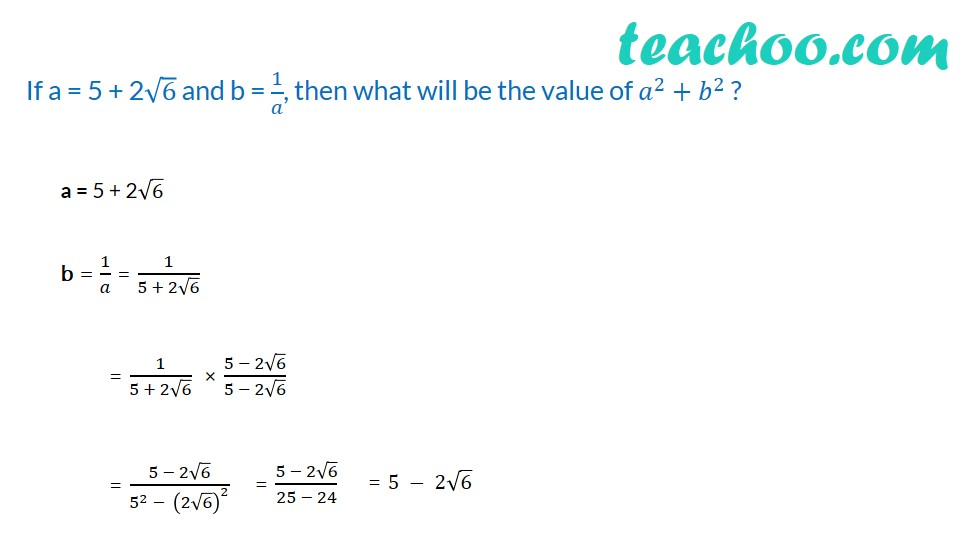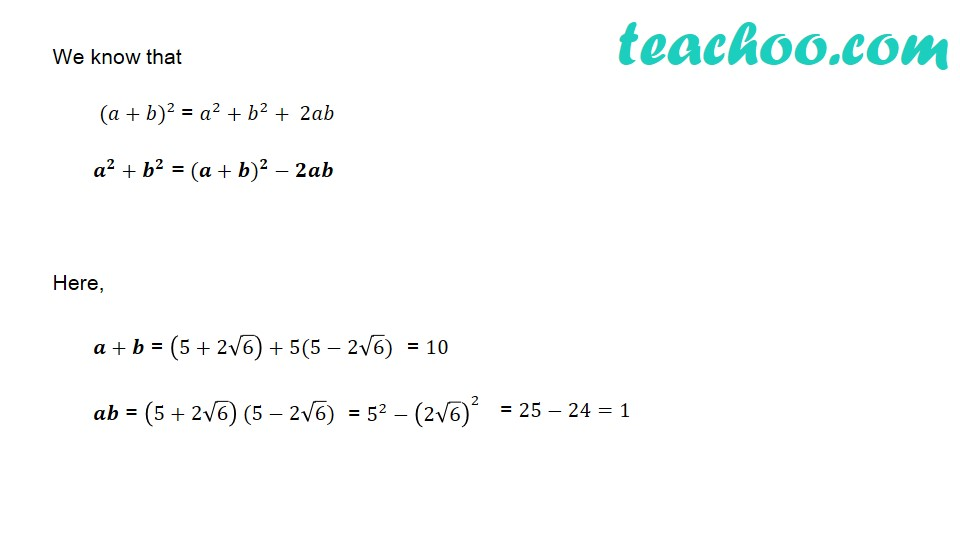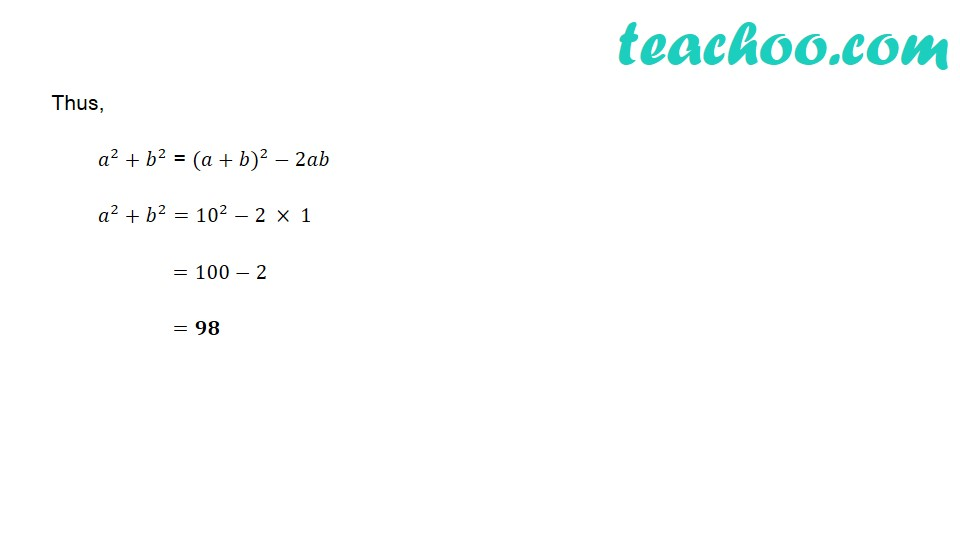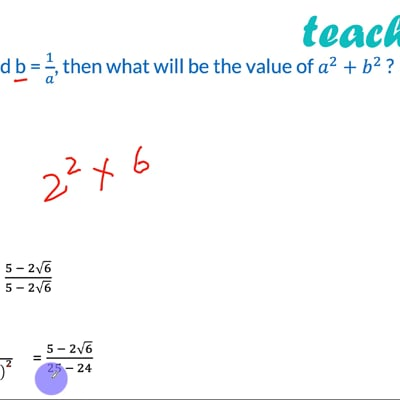Rationalising

Chapter 1 Class 9 Number Systems
Concept wiseThis video is only available for Teachoo black users

Introducing your new favourite teacher - Teachoo Black, at only ₹83 per month

### Transcript

If a = 5 + 2√6 and b = 1/𝑎, then what will be the value of 𝑎^2+𝑏^2 ? a = 5 + 2√6 b = 1/𝑎 = 1/(5 + 2√6) = 1/(5 + 2√6) × (5 − 2√6)/(5 − 2√6) = (5 − 2√6)/(5^2 − (2√6)^2 ) = (5 − 2√6)/(25 − 24) We know that 〖(𝑎+𝑏)〗^2 = 𝑎^2+𝑏^2+ 2𝑎𝑏 𝒂^𝟐+𝒃^𝟐 = 〖(𝒂+𝒃)〗^𝟐 − 𝟐𝒂𝒃 Here, 𝒂+𝒃 = (5+2√6)+5(5−2√6) 𝒂𝒃 = (5+2√6) (5−2√6) Thus, 𝑎^2+𝑏^2 = 〖(𝑎+𝑏)〗^2 − 2𝑎𝑏 𝑎^2+𝑏^2=〖10〗^2−2 × 1 =100−2 =𝟗𝟖